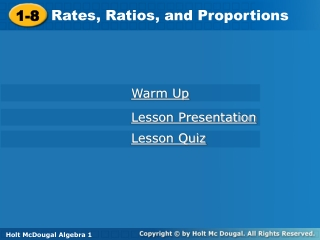DownloadDownload Presentation1-8

# 1-8

Download Presentation## 1-8

- - - - - - - - - - - - - - - - - - - - - - - - - - - E N D - - - - - - - - - - - - - - - - - - - - - - - - - - -
##### Presentation Transcript

1. Rates, Ratios, and Proportions 1-8 Holt Algebra 1 Warm Up Lesson Presentation Lesson Quiz Holt McDougal Algebra 1

2. Warm Up Solve each equation. Check your answer. 1. 6x = 36 2. 3. 5m = 18 4. 5. 8y =18.4 Multiply. 6. 7. 6 48 3.6 –63 2.3 7 10

3. Objectives Write and use ratios, rates, and unit rates. Write and solve proportions.

4. A ratio is a comparison of two quantities by division. The ratio of a to b can be written a:b or , where b ≠ 0. Ratios that name the same comparison are said to be equivalent. A statement that two ratios are equivalent, such as , is called a proportion.

5. Example 1: Using Ratios The ratio of the number of bones in a human’s ears to the number of bones in the skull is 3:11. There are 22 bones in the skull. How many bones are in the ears? There are 6 bones in the ears.

6. Check It Out! Example 1 The ratio of games won to games lost for a baseball team is 3:2. The team has won 18 games. How many games did the team lose? 12 losses

7. A rate is a ratio of two quantities with different units, such as Rates are usually written as unit rates. A unit rate is a rate with a second quantity of 1 unit, such as or 17 mi/gal. You can convert any rate to a unit rate.

8. Example 2: Finding Unit Rates Raulf Laue of Germany flipped a pancake 416 times in 120 seconds to set the world record. Find the unit rate. Round your answer to the nearest hundredth. The unit rate is about 3.47 flips/s.

9. Check It Out! Example 2 Cory earns \$52.50 in 7 hours. Find the unit rate. 7.5 = x The unit rate is \$7.50.

10. Example 3A: Using Dimensional Analysis A fast sprinter can run 100 yards in approximately 10 seconds. Use dimensional analysis to convert 100 yards to miles. Round to the nearest hundredth. (Hint: There are 1760 yards in a mile.) 100 yards is about 0.06 miles.

11. Example 3B: Using Dimensional Analysis A cheetah can run at a rate of 60 miles per hour in short bursts. What is this speed in feet per minute? The speed is 5280 feet per minute.

12. Check It Out! Example 3 A cyclist travels 56 miles in 4 hours. Use dimensional analysis to convert the cyclist’s speed to feet per second? Round your answer to the nearest tenth, and show that your answer is reasonable. The speed is about 20.5 feet per second.

13. Example 4: Solving Proportions Solve each proportion. A. B. m = 15 24 = y

14. Check It Out! Example 4 Solve each proportion. A. B. y = −20 g = 5.75

15. A scale is a ratio between two sets of measurements, such as 1 in:5 mi. A scale drawingor scale model uses a scale to represent an object as smaller or larger than the actual object. A map is an example of a scale drawing.

16. Example 5A: Scale Drawings and Scale Models A contractor has a blueprint for a house drawn to the scale 1 in: 3 ft. A wall on the blueprint is 6.5 inches long. How long is the actual wall? x = 19.5 The actual length of the wall is 19.5 feet.

17. Example 5B: Scale Drawings and Scale Models A contractor has a blueprint for a house drawn to the scale 1 in: 3 ft. One wall of the house will be 12 feet long when it is built. How long is the wall on the blueprint? The wall on the blueprint is 4 inches long.